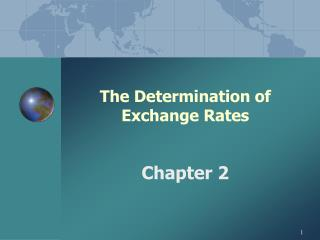DownloadDownload PresentationThe Determination of Exchange Rates

# The Determination of Exchange Rates

Télécharger la présentation## The Determination of Exchange Rates

- - - - - - - - - - - - - - - - - - - - - - - - - - - E N D - - - - - - - - - - - - - - - - - - - - - - - - - - -
##### Presentation Transcript

1. The Determination of Exchange Rates Chapter 2

2. CHAPTER 2THE DETERMINATION OF EXCHANGE RATES • CHAPTER OVERVIEW: • I. EQUILIBRIUM EXCHANGE RATES • II. ROLE OF CENTRAL BANKS • III. EXPECTATIONS AND THE ASSET MARKET MODEL

3. Part I. Equilibrium Exchange Rates • SETTING THE EQUILIBRIUM • EXCHANGE RATES • market-clearing prices that equilibrate the quantities supplied and demanded of a foreign currency.

4. Equilibrium Exchange Rates • How Americans Purchase German Goods • 1. Foreign Currency Demand • -derived from the demand for foreign country’s (German) goods, services, and financial assets by • locals (US). • e.g. The demand for German goods by Americans

5. Equilibrium Exchange Rates • 2. Foreign Currency Supply: • -derived from the foreign country’s demand for local (US) goods. • -They must convert their • currency to purchase. • e.g. German demand for US goods • means Germans convert Euro • to US \$in order to buy US • goods.

6. Equilibrium Exchange Rates • 3. Equilibrium Exchange Rate • occurs where the quantity supplied of Euro equals the • quantity demanded at a • specific local (US) price. • In this example the demand and supply for Euro is not only based on Germany and US

7. Equilibrium Exchange Rates • How Exchange Rates Change • 1. Increased demand • as more foreign goods are demanded, the price of the foreign currency in local currency increases and vice versa.

8. Equilibrium Exchange Rates • 2. Increased supply • as foreigners demand more local goods are, the price of the local currency in foreign currency increases and vice versa.

9. Equilibrium Exchange Rates • 3. Equilibrium Exchange Rate • adjusts based on the changes in the quantity supplied and the quantity demanded of a currency.

10. Equilibrium Exchange Rates • Home Currency depreciation • - Foreign currency becoming more valuable than the home currency. • - Conversely, the foreign currency’s value has appreciated against the • home currency.

11. Equilibrium Exchange Rates • 3. Calculating a Depreciation: • = (e0 - e1)/ e1 • where e0 = old currency value • e1 = new currency value

12. Equilibrium Exchange Rates • Currency Appreciation • = (e1 - e0)/ e0 • where e0 = old currency value • e1 = new currency value

13. Equilibrium Exchange Rates • EXAMPLE: Euro Appreciation • If the dollar value of the Euro goes from \$0.84 (e0) to \$0.89 (e1), then the Euro has appreciated by • (.89 - .84)/ .84 = 5.95%

14. Equilibrium Exchange Rates • EXAMPLE: US\$ Depreciation • We use the first formula, • (e0 - e1)/ e1 • substituting • (.84 - .89)/ .89 = - 5.62% • the US\$ depreciation.

15. Equilibrium Exchange Rates • D. FACTORS AFFECTING EXCHANGE RATES: • 1. Inflation rates • 2. Interest rates • 3. GNP growth rates • Of the two countries

16. EXPECTATIONS • Role of Expectations : • -Currency is a financial asset • -Exchange rate is simple relationship of two financial assets • -Expectations about the future plays an important role in determining the current value

17. EXPECTATIONS • Demand for Money and Currency Values: • Asset Market Model • A. Exchange rates reflect the supply of and demand for foreign-currency denominated assets.

18. EXPECTATIONS • B. Soundness of a Nation’s Economic Policies • A nation’s currency tends to strengthen with sound economic policies. • Strength of the economy is reflected on the currency.

19. EXPECTATIONS • EXPECTATIONS AND CENTRAL BANK BEHAVIOR • - exchange rates are also influenced by expectations of central bank behavior.

20. EXPECTATIONS • A. Central Bank Reputations • B. Central Bank Independence • C. Currency Boards

21. THE ROLE OF CENTRAL BANKS • V. FUNDAMENTALS OF CENTRAL BANK INTERVENTION • A. Role of Exchange Rates: • LINKS BETWEEN • THE DOMESTIC AND THE • WORLD ECONOMY

22. THE ROLE OF CENTRAL BANKS • B. IMPACT OF EXCHANGE RATE CHANGES • 1. Appreciation: • -domestic prices increase • relative to foreign prices. • -Exports: less competitive • Imports: more attractive

23. THE ROLE OF CENTRAL BANKS • 2. Currency Depreciation • - domestic prices fall relative to foreign prices. • - Exports: more price competitive. • - Imports: less attractive

24. THE ROLE OF CENTRAL BANKS • C. Foreign Exchange Market Intervention • 1. Definition: the official purchases and sales of currencies through the central bank to influence the home exchange rate.

25. THE ROLE OF CENTRAL BANKS • Intervention : • -Sterilized, by open market operations • -Unsterilized • Effectiveness of intervention